from IPython.core.display import HTML



# Script 9: miscellaneous¶

### Basic introduction to regular expressions¶

So called regular expressions describe patterns which can be used to search strings.

Such a pattern is a string, but some characters as .[]?+*()^ have special meanings.

Python has the re module to work with regular expressions, the function re.search looks for the first occurence of the pattern in the given string:

import re

sequence = "ATGCATGC"
pattern = "GCA"         # pattern withouth special character !

match = re.search(pattern, sequence)
if match != None:
print("first match:", match.group(), match.start(), match.end())
else:
print("no match")

first match: GCA 2 5


re.search returns either None or a "match object". As you can see in the example above this object has some methods to give you more information about the match.

The special symbol . encodes "any character". So you can see that GC. matches GCA:

sequence = "ATGCATGC"
pattern = "GC."

match = re.search(pattern, sequence)
if match != None:
print("first match:", match.group(), match.start(), match.end())
else:
print("no match")

first match: GCA 2 5


[...] means "exactly one of the characters between the brackets":

print(re.search("GC[ATG]", "GCAG") != None)
print(re.search("GC[ATG]", "GCTG") != None)
print(re.search("GC[ATG]", "GCCG") != None)

True
True
False


To find all occurences you can use finditer:

for match in re.finditer("GC[AT]", "GCAGAAGCTGCC"):
print(match.group(), match.start(), match.end())

GCA 0 3
GCT 6 9

for match in re.finditer("GC.", "GCAGAAGCTGCC"):
print(match.group(), match.start(), match.end())

GCA 0 3
GCT 6 9
GCC 9 12


The expression | means "either or":

print(re.search("A(bcd|BCD)E", "ABCDE") != None)
print(re.search("A(bcd|BCD)E", "AbcdE") != None)
print(re.search("A(bcd|BCD)E", "ABcdE") != None)

True
True
False


### Exercises¶

• reproduce the previous examples and play with them

## More about range¶

range accepts zero to two arguments:

• range(n) counts from 0 to n - 1.
• range(m, n) counts from m to n - 1
• range(m, n, k) counts from m to n - 1 with step size k
for i in range(2, 11, 3):
print(i)

2
5
8

for i in range(6, 3, -1):
print(i)

6
5
4


We now combine slicing and range with three arguments to split a sequence into codons:

def split_codons(sequence):
codons = []
for start in range(0, len(sequence), 3):
codon = sequence[start:start + 3]
codons.append(codon)
return codons

print(split_codons("ATGCATGCA"))

['ATG', 'CAT', 'GCA']


## Exercises¶

1. Reproduce the examples above

## Functions with multiple return values¶

Functions may compute more than one result. In this case you have to list the values separated by , after return:

def sum_and_diff(a, b):
return a + b, a - b

x, y = sum_and_diff(10, 3)
print(x, y)

13 7


You can see above that we have to use x, y = sum_and_diff(10, 3) to receive both return values in given order. x will be the value of a + b and y will be a - b.

Python provides some shortcuts for common algebraic operations:

• x += m is the same as x = x + m
• x -= m is the same as x = x - m
• x *= m is the same as x = x * m
• x /= m is the same as x = x / m

Here is an example which combines algebraic updates and multiple return values:

def statistics(li):
count = 0
acc = 0
for element in li:
count += 1
acc += element
return count, acc, acc / count

count, sum, average = statistics([1, 2, 3, 4, 5, 6])
print("counted", count, "numbers having sum", sum, "and average", average)

counted 6 numbers having sum 21 and average 3.5


## Exercise block 3¶

1. Reproduce the examples above
2. Write a function which returns minimum and maximum of a given list of numbers

## More about print¶

We already introduced the form print(..., file=fh) to write to a file instead displaying the output on the console. Beyond that print has other (so called) named arguments.

Before we introduce other named arguments, we state:

• every print will automatically output a \n after execution, so the next print will display output on a new line
• a plain print() creates an empty line
• print with mutliple arguments will separate them by a single space " ":

You can observe this in the following example:

print(3)
print()
print(1, 2, 3)

3

1 2 3


To modify some of these properities the named parameters sep and end come into play. sep allows other separators than a single blank character:

print(1, 2, 3, sep=", ")

1, 2, 3


And end allows to override the default \n to avoid the line break:

print(1, 2, 3, end=" ")
print(4)
print(1, 2, 3, end="")
print(4)

1 2 3 4
1 2 34


We can use this to print a multiplication table:

def print_table(up_to):
for row in range(1, up_to + 1):
for col in range(1, up_to + 1):
cell_value = row * col
print(cell_value, end=" ")
print()

print_table(9)

1 2 3 4 5 6 7 8 9
2 4 6 8 10 12 14 16 18
3 6 9 12 15 18 21 24 27
4 8 12 16 20 24 28 32 36
5 10 15 20 25 30 35 40 45
6 12 18 24 30 36 42 48 54
7 14 21 28 35 42 49 56 63
8 16 24 32 40 48 56 64 72
9 18 27 36 45 54 63 72 81


The table looks a bit ugly, so we add a leading space for numbers with one digit:

def print_pretty_table(up_to):
for row in range(1, up_to + 1):
for col in range(1, up_to + 1):
cell_value = row * col
if cell_value < 10:
print(" ", end="")
print(cell_value, end=" ")
print()

print_pretty_table(9)

 1  2  3  4  5  6  7  8  9
2  4  6  8 10 12 14 16 18
3  6  9 12 15 18 21 24 27
4  8 12 16 20 24 28 32 36
5 10 15 20 25 30 35 40 45
6 12 18 24 30 36 42 48 54
7 14 21 28 35 42 49 56 63
8 16 24 32 40 48 56 64 72
9 18 27 36 45 54 63 72 81


## String interpolation¶

The expression you see between the brackets of print is called "string interpolation":

def greet(name):
print("hi %s how do you do" % name)

greet("bart simpson")

hi bart simpson how do you do


Explanation: The %s is a place holder, and when Python evaluates "hi %s how do you do" % name the value of name will be inserted at this position.

For multiple place holders in the template you have to use round brackets after %, place holders are filled with the given values in order:

def print_sum(a, b):
print("%s plus %s is %s" % (a, b, a + b))

print_sum(3, 4)

3 plus 4 is 7


%s is one of many possible place holders. Another place holder has the form %.nf where n is a number and f is fixed, formats a number with n digits after the decimal point:

import math

# print only two numbers after decimal point
print("pi with two digits is %.2f" % math.pi)

pi with two digits is 3.14

print("%.1f" % 3)

3.0


The form %m.nf formats with n numbers after the decimal point and pads spaces from the left so that the full result is at least m characters wide:

print("%5.2f" % math.pi)
print("%5.2f" % 11.3)
print("%5.2f" % 121.3)

 3.14
11.30
121.30


For integer numbers you can use %nd for padding up to n characters:

print("%3d" % 4)
print("%3d" % 44)

  4
44


And e and variations print in scientific notation:

print("%e" % math.pi)
print("%.2e" % math.pi)
print("%10.2e" % math.pi)

3.141593e+00
3.14e+00
3.14e+00


## Exercise block 4¶

1. Reproduce the examples above and try to understand how the multiplication table examples work !
2. Can you rewrite print_pretty_table without the if by using string interpolation instead ?
3. Write a function print_triangle(n) which displays a symmetric triangle of height n. Assume that n is an odd number. So print_triangle(5) displays

    *
***
*****
*******
*********

For common operations on lists Python provides some convenience functions. They replace some computations we exercised up to now.

To compute the maximum or minimum of a given list max and min functions exist:

print(max([2, 3, 1]))

3

print(min([2, 3, 1]))

1


Beyond that sorted computes a sorted list from a given list:

print(sorted([3, 1, 2]))

[1, 2, 3]


Many tasks with lists as transforming or filtering a list can be expressed by so called list comprehensions. Here are a few examples:

numbers = range(10)
squared_numbers = [n ** 2 for n in numbers]
print(squared_numbers)

[0, 1, 4, 9, 16, 25, 36, 49, 64, 81]


This is a shortcut for:

numbers = range(10)
squared_numbers = []
for n in numbers:
squared_numbers.append(n ** 2)
print(squared_numbers)

[0, 1, 4, 9, 16, 25, 36, 49, 64, 81]


A more complex list comprehension is:

numbers = range(10)
squared_even_numbers = [n ** 2 for n in numbers if n % 2 == 0]
print(squared_even_numbers)

[0, 4, 16, 36, 64]


This is an shortcut for:

numbers = range(10)
squared_even_numbers = []
for n in numbers:
if n % 2 == 0:
squared_even_numbers.append(n ** 2)
print(squared_even_numbers)

[0, 4, 16, 36, 64]


Sometimes we want to iterate over two iterables at the same time. This can be implemented with the zip function:

values = [1, 2, 1, 2, 1, 2]
groups = [0, 0, 0, 1, 1, 1]

for v, g in zip(values, groups):
print("value", v, "is in group", g)

value 1 is in group 0
value 2 is in group 0
value 1 is in group 0
value 2 is in group 1
value 1 is in group 1
value 2 is in group 1


When "zipping" the shorter iterable determines when looping ends:

for i, j in zip(range(4), range(2)):
print(i)

0
1


To retrieve an iteraton index enumerate helps:

with open("amino_acids.csv", "r") as fh:
for i, line in enumerate(fh):
print("line", i, "has length", len(line.rstrip()))
if i >= 10:
break

---------------------------------------------------------------------------
FileNotFoundError                         Traceback (most recent call last)
<ipython-input-34-2b65d046c1ff> in <module>()
----> 1 with open("amino_acids.csv", "r") as fh:
2     for i, line in enumerate(fh):
3         print("line", i, "has length", len(line.rstrip()))
4         if i >= 10:
5             break

FileNotFoundError: [Errno 2] No such file or directory: 'amino_acids.csv'

## Exercise block 5¶

1. Reproduce the examples above
2. Rewrite the script for grouping with dictionaries using zip to avoid indexed access with [].
3. (optional) Write a script which creates a plain FASTA text file which contains the 3 longest sequences a given input FASTA file. It is ok if you write the sequence as one line in the output file. In case of duplicate lengthts, like 10, 10, 9, 9, 8 you can write the top 4 sequences from this list. Hint: create a list with the lengths of the sequences, sort it and find so a threshold value for filtering the given sequences.

## Basic Plotting¶

The next section demonstrates how to plot diagrams with Python. The used library http://matplotlib.org/ is comprehensive, we just can show a few examples here. Look at the gallery http://matplotlib.org/gallery.html to see what this library offers.

1. Check if import pylab works
2. If you see an ImportError exception read in 02_introduction_pycharm in section "Install extra libraries" how to install the missing library

### Instructions:¶

• reproduce the following examples on your computer
• play with the examples, I included some suggestions as comments in the code snippets
import pylab
import random

x_values = []
for i in range(1000):
random_number = random.normalvariate(0, 100.0) # mean 0.0 std deviation 100.0
x_values.append(random_number)

"""
play with the parameters "bins" and "color" !!!
"""
pylab.hist(x_values, bins=20, color="b")
pylab.show()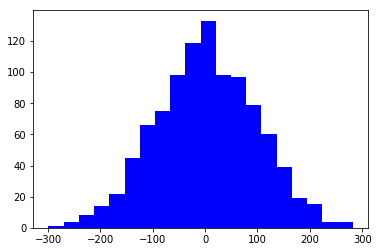import pylab
import random

x_values = []
y_values = []
for i in range(1000):
x = random.normalvariate(0, 100.0) # mean 0.0 std deviation 100.0
y = x + random.normalvariate(0.0, 30.0)

x_values.append(x)
y_values.append(y)

"""
what happens if you use "b*" instead of "r." ??
"""
pylab.plot(x_values, y_values, "r.")   # red dots
pylab.show()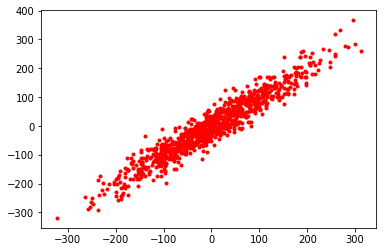Now we group four plots:

# set the overall size of the next plot in (width, height) inches
pylab.figure(figsize=(18, 4))

pylab.subplot(1, 4, 1)   # one row, four columns, plot number 1
pylab.plot(x_values, 'y')

pylab.subplot(1, 4, 2)
pylab.hist(x_values, bins=20, color="r")

pylab.subplot(1, 4, 3)
pylab.hist(y_values, bins=20, color="g")

pylab.subplot(1, 4, 4)
pylab.plot(x_values, y_values, "b.")

pylab.show()

"""suggestions for playing:
- arange the plots in two rows and two columns
- play with the figsize paramter above
- choose "random_hist.pdf" as a file name below !
"""

# we can save this plot to disk, you should find the file in PyCharms project folder afterwards.
# If you want to save only, you can ommit the "pylab.show()" above !
pylab.savefig("random_hist.png")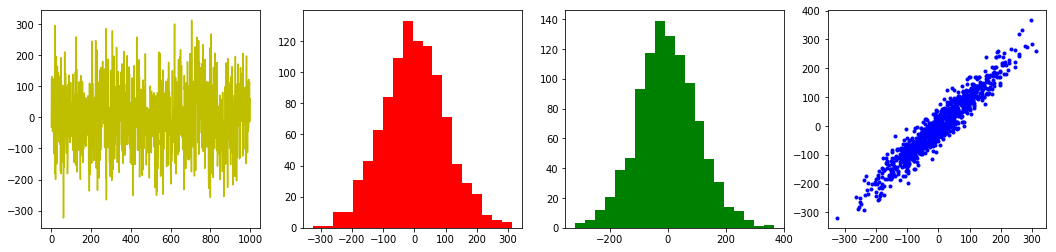## Numpy¶

numpy provides data containers for vectors and matrices:

import numpy

x = numpy.arange(0.0, 6.0, 0.25)  # similar to range: arguments are start, excluive upper limit, stepsize
print(repr(x))

array([0.  , 0.25, 0.5 , 0.75, 1.  , 1.25, 1.5 , 1.75, 2.  , 2.25, 2.5 ,
2.75, 3.  , 3.25, 3.5 , 3.75, 4.  , 4.25, 4.5 , 4.75, 5.  , 5.25,
5.5 , 5.75])


numpy vectors are more handy the usual Python lists, so we can apply a single numpy function elementwise to a vector like this:

# to apply a function to all elements of a vector:
y = numpy.sin(x)


(For large vectors this is much faster than performing the same operation with a for loop over lists)

print(y)  # without repr it prints as a regular list, but is still is an numpy array !

[ 0.          0.24740396  0.47942554  0.68163876  0.84147098  0.94898462
0.99749499  0.98398595  0.90929743  0.7780732   0.59847214  0.38166099
0.14112001 -0.10819513 -0.35078323 -0.57156132 -0.7568025  -0.89498936
-0.97753012 -0.99929279 -0.95892427 -0.85893449 -0.70554033 -0.50827908]

y2 = numpy.sin(.75 * x)
pylab.plot(x, y, label="sine")
pylab.plot(x, y2, label="slower sine")
pylab.title("two sines")
pylab.legend()
pylab.show()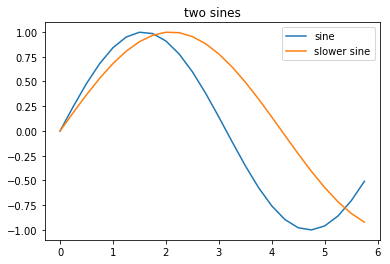### Heatmap¶

import numpy
import pylab

# we use the next statement to adapt the output format if we print numpy data)
numpy.set_printoptions(precision=4, linewidth=100)

# we create a 10 x 10 matrix with random values in range 0.0 to 1.0:
matrix = numpy.random.random((10,10))
print(matrix)

pylab.figure(figsize=(5, 5))  # so we enforce equal sized axes, remove this line and see the difference !
pylab.pcolor(matrix, cmap=pylab.cm.hot)

# play with other colormaps ! (see http://matplotlib.org/examples/color/colormaps_reference.html)
pylab.show()

[[0.8745 0.2913 0.6812 0.8116 0.5561 0.3211 0.3627 0.7007 0.1928 0.4708]
[0.8983 0.4427 0.4127 0.5456 0.4444 0.0888 0.3685 0.0462 0.9977 0.3371]
[0.037  0.2489 0.7584 0.1951 0.5764 0.8399 0.9789 0.0705 0.7282 0.3371]
[0.7199 0.2629 0.9994 0.3374 0.0374 0.8804 0.3298 0.941  0.0133 0.0496]
[0.1104 0.1201 0.1918 0.3    0.0212 0.7441 0.8856 0.2191 0.6653 0.8743]
[0.9103 0.8107 0.9036 0.5548 0.7513 0.379  0.0141 0.5196 0.3581 0.1641]
[0.0494 0.0132 0.8235 0.1342 0.4745 0.5146 0.6767 0.7864 0.2055 0.726 ]
[0.6917 0.3839 0.0767 0.4964 0.3553 0.8096 0.5948 0.5142 0.8777 0.3139]
[0.1228 0.7978 0.5501 0.8882 0.9072 0.7104 0.7393 0.1844 0.5129 0.8373]
[0.8465 0.7171 0.9909 0.7896 0.4152 0.5469 0.3418 0.4266 0.7667 0.1618]]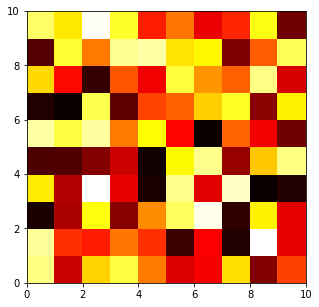Try to identify the colors with cell numbers above, what do you observe ???

## Exercise block 5¶

1. Reproduce all examples above, play with them !

2. Plot two histograms in a row: a histogram of the sequence lengths in a given the FASTA file and another histrogram of the AG content of the sequences.